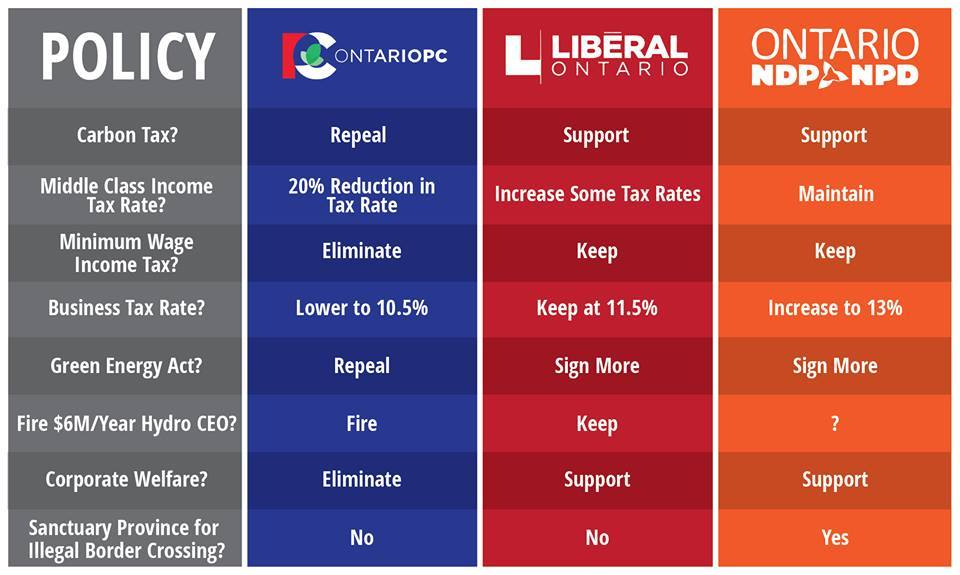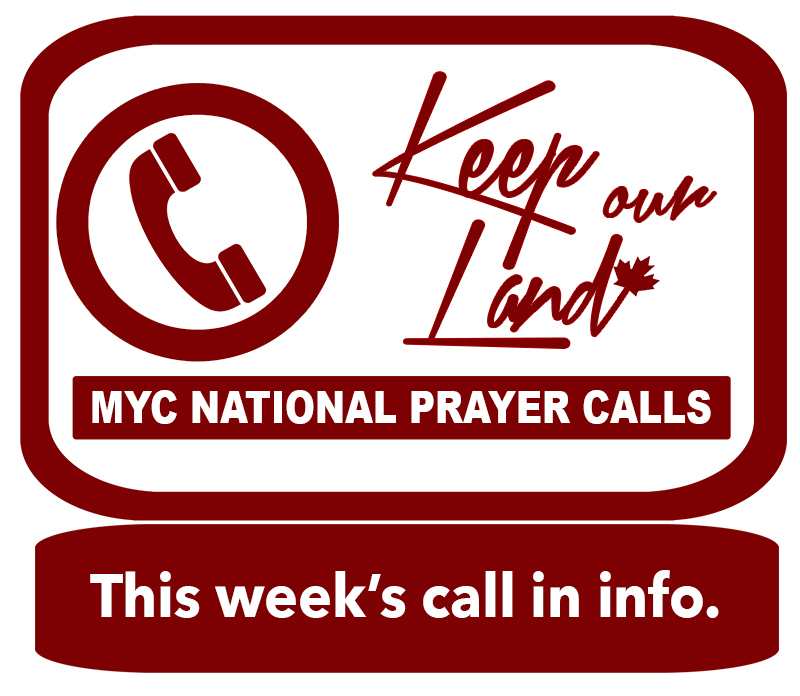Like   Tweet   Pin   +1   in
 /* styles */ SPECIAL NOTICE REGARDING MARIJUANA BILL: Please also keep the Senate in prayer. They are expected to vote on the marijuana legislation on Thursday as well. There is still time to contact our Senators to share your heart with them about this issue. Click here to find out more.
 table div table+table+table div table{width:100%;padding:0}table div table+table+table div table img{width:96.23%;padding:0;float:none}table div table+table+table div table td{width:100%;padding:0 1.88% 18px}/* styles *//* styles */ In 2 days, on June 7th, Ontario will choose their new provincial government. This election will affect over 1/3 of the nation's population and will inevitably impact the rest of Canada. When Ontario's economy and legislation is healthy it could affect the whole nation because of example and because of transfer payments. The inverse is also true. No matter where you live, please join in praying for this important election. If you live in Ontario we want to encourage you to get out and vote. As mentioned last week, we strongly encourage you to vote for the party that will be the best stewards of the provinces finances as this affects almost all other issues. According to Lisa Raitt, currently Ontario pays a billion dollars a month to interest on it's debt. That could build a hospital a month. Management of provincial funds is a lead domino issue. Please also vote for the party which will stand for family values & parental rights. This is not a time to vote based on personality but based on policies that will best serve future generations. Our hope is not in government, it is in God. At the same time we recognize God has given us a command to be salt and light in our generation. Exercising our freedom to vote is one way we can influence our generation in a positive way. Jesus was both a man of prayer, and a man of action. Let us be the same. Thank you for your love for Ontario and participation at this important time. Below are a few resources to help you in your decision.
 table div table+table+table+table+table div table{width:100%;padding:0}table div table+table+table+table+table div table img{width:96.23%;padding:0;float:none}table div table+table+table+table+table div table td{width:100%;padding:0 1.88% 18px}/* styles */# ONTARIO VOTING RESOURCE

ELECTIONS ONTARIO // Click here to go to Elections Ontario and get all the info you need about where and how to vote on Thursday.

# WHERE THE PARTIES STANDtable div table+table+table+table+table+table+table+table div table td,table.module-7{width:100%;padding:0}table div table+table+table+table+table+table+table+table div table{width:100%;float:none;margin-left:auto;margin-right:auto;padding:0}table div table+table+table+table+table+table+table+table div table a{border:0 none;text-decoration:none}table div table+table+table+table+table+table+table+table div table img{width:100%!important;border:0 none;text-decoration:none}/* styles */
 table div table+table+table+table+table+table+table+table+table div table{width:100%;padding:0}table div table+table+table+table+table+table+table+table+table div table img{width:96.23%;padding:0;float:none}table div table+table+table+table+table+table+table+table+table div table td{width:100%;padding:0 1.88% 18px}/* styles */table div table+table+table+table+table+table+table+table+table+table div table{width:100%;padding:0}table div table+table+table+table+table+table+table+table+table+table div table img{width:96.23%;padding:0;float:none}table div table+table+table+table+table+table+table+table+table+table div table td{width:100%;padding:0 1.88% 18px}/* styles */table div table+table+table+table+table+table+table+table+table+table+table div table td,table.module-10{width:100%;padding:0}table div table+table+table+table+table+table+table+table+table+table+table div table{width:100%;float:none;margin-left:auto;margin-right:auto;padding:0}table div table+table+table+table+table+table+table+table+table+table+table div table a{border:0 none;text-decoration:none}table div table+table+table+table+table+table+table+table+table+table+table div table img{width:100%!important;border:0 none;text-decoration:none}/* styles */

We encourage you to check out our director, Faytene Grasseschi's, weekly show which is attacking key issues for our nation head on.

Visit faytene.tv for information on upcoming shows or to watch those which have already aired.

Shows have covered topics such as marijuana legislation, doctor conscience rights, first nations suicide, poverty, human trafficking, abortion harassment, sex education and more.

## AIRING TIMES

SUNDAY MORNINGS ON VISION //

6:30am PST / 7:30am MST / 8:30am CST/ 9:30am EST / 10:30am AST

SATURDAY AFTERNOONS ON DAYSTAR CANADA //

10:30am PST / 11:30am MST / 12:30pm CST/ 1:30pm EST / 2:30pm AST

SUNDAY NIGHTS ON DAYSTAR CANADA // 8:30 pm EST

5:30pm PST / 6:30pm MST / 7:30pm CST/ 8:30pm EST / 9:30pm AST

SUNDAY NIGHTS ON YES TV // 9:30 pm EST

Visit www.faytene.tv for even more informative programs on issues that are shaping the future of our nation.

 table div table+table+table+table+table+table+table+table+table+table+table+table+table div table td,table.module-12{width:100%;padding:0}table div table+table+table+table+table+table+table+table+table+table+table+table+table div table{width:100%;float:none;margin-left:auto;margin-right:auto;padding:0}table div table+table+table+table+table+table+table+table+table+table+table+table+table div table a{border:0 none;text-decoration:none}table div table+table+table+table+table+table+table+table+table+table+table+table+table div table img{width:100%!important;border:0 none;text-decoration:none}/* styles */
 table div table+table+table+table+table+table+table+table+table+table+table+table+table+table div table td,table.module-13{width:100%;padding:0}table div table+table+table+table+table+table+table+table+table+table+table+table+table+table div table{width:100%;float:none;margin-left:auto;margin-right:auto;padding:0}table div table+table+table+table+table+table+table+table+table+table+table+table+table+table div table a{border:0 none;text-decoration:none}table div table+table+table+table+table+table+table+table+table+table+table+table+table+table div table img{width:100%!important;border:0 none;text-decoration:none}/* styles */
 table div table+table+table+table+table+table+table+table+table+table+table+table+table+table+table div table td,table.module-14{width:100%;padding:0}table div table+table+table+table+table+table+table+table+table+table+table+table+table+table+table div table{width:100%;float:none;margin-left:auto;margin-right:auto;padding:0}table div table+table+table+table+table+table+table+table+table+table+table+table+table+table+table div table a{border:0 none;text-decoration:none}table div table+table+table+table+table+table+table+table+table+table+table+table+table+table+table div table img{width:100%!important;border:0 none;text-decoration:none}/* styles */
 table div table+table+table+table+table+table+table+table+table+table+table+table+table+table+table+table div table{width:100%;padding:0}table div table+table+table+table+table+table+table+table+table+table+table+table+table+table+table+table div table table{padding:0;float:left!important;width:60.566%!important}table div table+table+table+table+table+table+table+table+table+table+table+table+table+table+table+table div table table+table td,table div table+table+table+table+table+table+table+table+table+table+table+table+table+table+table+table div table td{padding-left:0;padding-right:0}table div table+table+table+table+table+table+table+table+table+table+table+table+table+table+table+table div table table td{padding-left:0;padding-right:20px}table div table+table+table+table+table+table+table+table+table+table+table+table+table+table+table+table div table table+table{float:left!important;width:39.434%!important}/* styles */
 table div table+table+table+table+table+table+table+table+table+table+table+table+table+table+table+table+table div table{width:100%;padding:0}table div table+table+table+table+table+table+table+table+table+table+table+table+table+table+table+table+table div table table{padding:0;float:left!important;width:52.264%!important}table div table+table+table+table+table+table+table+table+table+table+table+table+table+table+table+table+table div table table+table td,table div table+table+table+table+table+table+table+table+table+table+table+table+table+table+table+table+table div table td{padding-left:0;padding-right:0}table div table+table+table+table+table+table+table+table+table+table+table+table+table+table+table+table+table div table table td{padding-left:0;padding-right:20px}table div table+table+table+table+table+table+table+table+table+table+table+table+table+table+table+table+table div table table+table{float:left!important;width:47.736%!important}/* styles */
 table div table+table+table+table+table+table+table+table+table+table+table+table+table+table+table+table+table+table div table{width:100%;padding:0}table div table+table+table+table+table+table+table+table+table+table+table+table+table+table+table+table+table+table div table img{width:96.23%;padding:0;float:none}table div table+table+table+table+table+table+table+table+table+table+table+table+table+table+table+table+table+table div table td{width:100%;padding:0 1.88% 18px}/* styles */## Bi-Weekly Prayer Call:

MY Canada is hosting national prayer conference calls every second Tuesday at noon EST.